# Labeling labeled objects

A post in comp.soft-sys.matlab this week (see the third post in this thread) asked how to display graphically the numerical labels associated with each labeled object. In this blog I'll show a couple of ways to approach that task.

First, let's back up a bit. We're talking about labeling connected groups of pixels in binary images. Here's an example, starting with the grayscale image coins.png.

I = imread('coins.png');
imshow(I)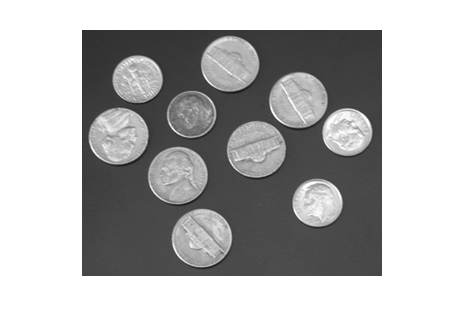Threshold to form a binary image.

bw = im2bw(I, graythresh(I));
imshow(bw)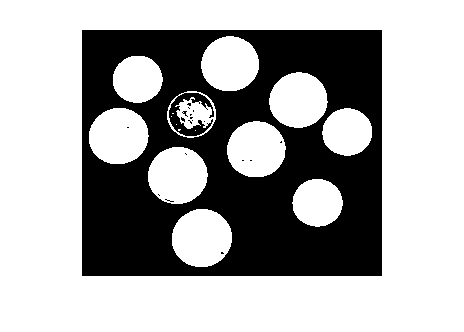Fill in the holes.

bw2 = imfill(bw, 'holes');
imshow(bw2)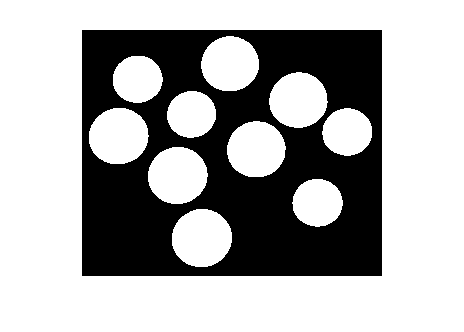You can easily see that there are 10 objects in the image. But to start doing geometric measurements, we have to be able to identify which foreground pixels belong to which object. That's what bwlabel does.

L = bwlabel(bw2);

L is a label matrix. It's the same size as bw2. It contains the value 0 in each location that's a background pixel. It contains positive integer values in locations corresponding to a labeled foreground object. For example, to see the eighth object, you just determine all the elements of L that equal 8:

imshow(L == 8)
title('Object 8')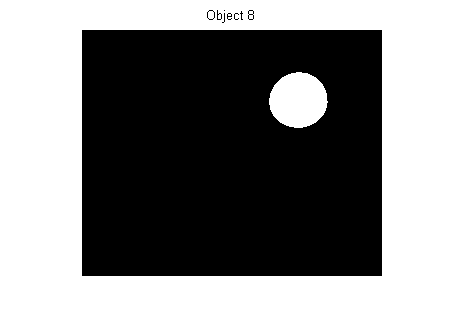Getting back to the original question - How can we graphically display each object's label? One way is to compute the centroid of each object, and then superimpose text strings on top of the image at the centroid locations. Here's one implementation:

s = regionprops(L, 'Centroid');
imshow(bw2)
hold on
for k = 1:numel(s)
c = s(k).Centroid;
text(c(1), c(2), sprintf('%d', k), ...
'HorizontalAlignment', 'center', ...
'VerticalAlignment', 'middle');
end
hold off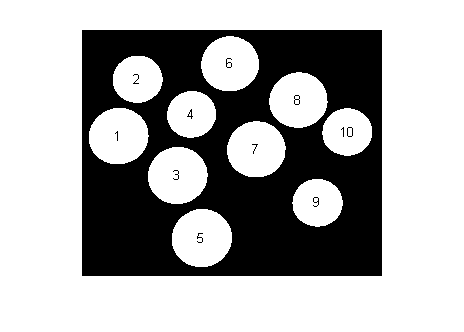My second method is more interactive. It uses a fairly new MATLAB feature: Data cursors.

If you haven't seen MATLAB data cursors before, here's a screen shot showing what happens when you click on a plot in data cursor mode.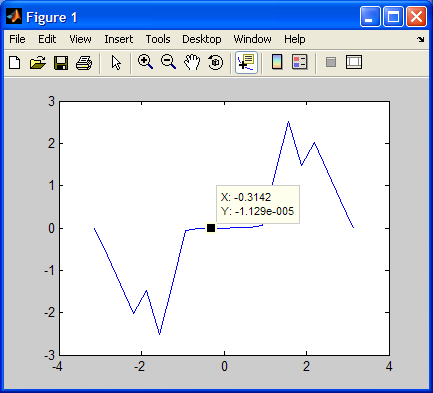Data cursors work for images, too: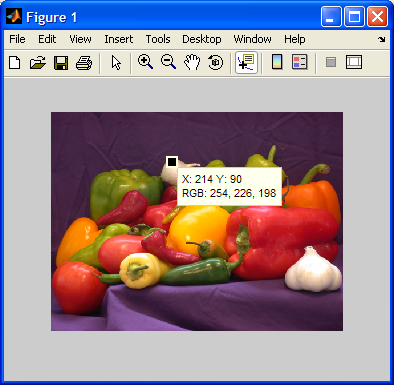With a modest bit of programming (see Customizing Data Cursor Text), you can customize the data cursor strings. Here, we just want the object label to be displayed. I wrote a little function called display_label_matrix that does just that. It's just a dozen lines of code. Here's what it looks like for the coins example: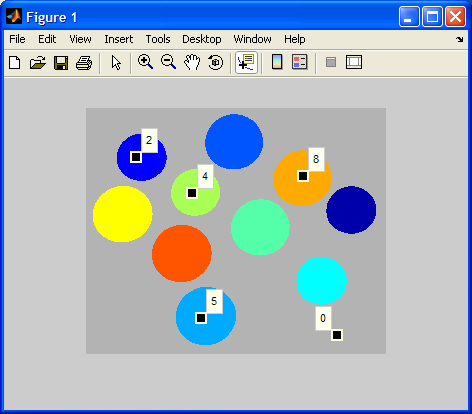And here's the code:

type display_label_matrix
function display_label_matrix(L)
% display_label_matrix Display label matrix with custom data cursors
% display_label_matrix(L) displays the label matrix L as colored objects on
% a gray background.  It also installs a custom data cursor handler in the
% figure.  In data cursor mode, clicking on an object displays a data
% cursor containing the object's number.

% Steve Eddins
% Copyright 2006 The MathWorks, Inc.
% $Revision: 1.1$  $Date: 2006/11/17 19:11:30$

rgb = label2rgb(L, 'jet', [.7 .7 .7], 'shuffle');
h = imshow(rgb);

% Store the label matrix so that the custom data cursor function has access
% to it.
setappdata(h, 'LabelMatrix', L);

% Enable the figure data cursor mode, and use our own custom data cursor
% strings.
dcm = datacursormode(ancestor(h, 'figure'));
set(dcm,'Enable','on', ...
'Updatefcn', @dataCursorText);

%==========================================================================
function output_text = dataCursorText(obj, event_obj)
% Respond to a user click in data cursor mode by displaying the label
% number of the pixel that was clicked on.

h_image = get(event_obj, 'Target');
pos = get(event_obj, 'Position');
L = getappdata(h_image, 'LabelMatrix');
clicked_label = L(round(pos(2)), round(pos(1)));
output_text = sprintf('%d', clicked_label);
%--------------------------------------------------------------------------



Give it a try and let me know what you think.

Published with MATLAB® 7.3

|